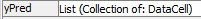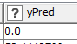# Working with List Columns from R Snippet

I have an R Snippet where I am using `minpack` to fit a curve for each row of the table. x and y values for each prediction are in 2 list columns, which after a bit of fiddling I figured I can use as follows:

``````## Collection columns come in protected as AsIs types by call to I(x)
## This function unwraps back to their actual type
## From https://stackoverflow.com/a/12866609/6076839
unAsIs <- function(X) {
if("AsIs" %in% class(X)){
class(X) <- class(X)[-match("AsIs", class(X))]
}
X
}

## Here we set up an output dataframe for the fitting results
res <- ...

## Loop over rows fitting for each row
for(row in 1:nrow(knime.in)) {
x<-unlist(unAsIs(knime.in[row,"x-col"]))
y<-unlist(unAsIs(knime.in[row,"y-col"]))

eqn <- y~I(...)
out <- nlsLM(eqn,
data=data.frame(x,y),
start=list(...),
control=nls.control(maxiter=500, tol=1e-10, minFactor=1/(1024*1024), printEval=TRUE),
trace=TRUE
)
sum <- summary(out)
## Calculated the values of y predicted from the fitted values
yPred <-predict(out,x))
## Add the fit parameters to the table
res[row, ]<-c(....)
}
##We lose the collection column types, so instead propagate rowIDs and join back
rownames(res) <- rownames(knime.in)
knime.out <- res
``````

There are two issues here:

1. I would like to add the value of yPred to the output table as a collection column, but not matter what I try I dont manage to add the list, only a ‘0’ in each output row (`yPred` is an `AsIs` which can be unwrapped to a `list()` - I’ve then tried `unlist(unAsIs(yPred))` and various other combinations - none of which work) - can anyone tell me how to do this?
2. The second issue is hinted at in the final 3 lines - any incoming collection columns lose their type information, so for example `x-col` is a List of Doubles in the incoming table, but if I pass the incoming columns through using `knime.out <- cbind(knime.in,res)` then they become an untyped List of DataCell. Is there a workaround for this, other than my approach here, which is to propagate the RowIDs from the input table, drop the incoming columns, and use a `Joiner` node after the snippet to reconnect the incoming table columns?

Thanks

Steve

Of course, having posted the question, I have a partial answer, based loosely on the answer to a similar question on StackOverflow -

``````	yPred <-list(list(unAsIs(predict(out,x))))
res[row,] <- c(...., yPred)
``````

(That’s a bit unintuitive, but it makes sense with R’s vector recycling that the list has to be re-wrapped in another list of one member - the list of interest)

However, this again runs into the untyped collection column in the output table problem:And indeed `UnGroup`-ing the column shows that KNIME doesn’t know what’s in the collection:The only way I can see to fix is to add an index column, ungroup, correct the type of yPred to Double, and then regroup on the index, which feels somewhat cumbersome, or to pass through a Java Snippet, with the input `yPred` set as `Array of String (toString())`, and the snippet:

``````out_yPred = Arrays.stream(c_yPred)
.map(str -> Double.parseDouble(str)).toArray(Double[]::new);
``````

Is there a better way?

Steve

@s.roughley maybe you could amend your example with a working dataset so one might be able to test it.

2 Likes

Sure. Here’s a simple toy example fitting several rows to their own `y = mx + c` simple straight lines, which hopefully shows all the issues described (and the partial solutions / workarounds in case anyone else is in the same or similar situation with handling collection columns in the R snippet and related nodes)

Curve fit forum question.knwf (20.9 KB)

Steve

2 Likes

@s.roughley well indeed a strange thing this loss of type.

I found one thing that would preserve the type if you save the data from within the R node as a parquet file and then read it back (it works on a Mac at least). Not the most elegant way.

2 Likes

Thanks @mlauber71
I guess for complicated tables then that would work better than my hack which would need to be applied for every collection column output.

I did wonder whether changing to the columnar storage which is based on Apache Parquet would work based on your answer, but it looks like it doesn’t! I guess that means that there is something about the way R and KNIME transfer the data between themselves which `write-parquet` bypasses…

Steve

2 Likes

I tried to experiment with the two data transfer methods from the R snippet but to no avail. I checked the parquet file from within R(Studio) and it seems all the list elements in the parquet file are seen as double (the code is in the /data/ subfolder). I have no idea what is the difference.

I also tried to use ARFF but it would not work with these lists.

2 Likes